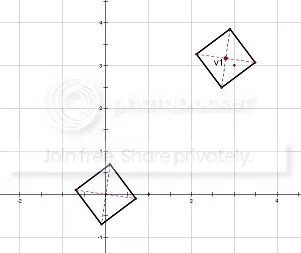Categories

# Encouraging Persistence Through Contest Problems, Part 2

In my last post, we looked at an AMC-12 problem of moderate difficulty, but with an premise that could be understood by many.  This time, we’ll take a look at a problem which delves into more abstract concepts, and explore how technology can allow students to consider solutions.  The following problem was question #23 from this year’s AMC-12.

Let S be the square one of whose diagonals has endpoints (0.1, 0.7) and (-0.1, -0.7).  A point v = (x,y) is chosen uniformly at random over all real numbers x and y such that x is between 0 and 2012, inclusive, and y is between 0 and 2012, inclusive.  Let T(v) be a translated copy of S centered at v.  What is the probability that the square region determined by T(v) contains exactly two points with integer coordinates in its interior?

In this year’s contest, where over 72,000 students participated, this question was answered correctly by only 4.5% of students, and was left blank by 81.6%.

Tomorrow morning, write this question in its entirety on a side board, and observe student reactions.  How many students begin to sketch the square described in the first sentence?  How many ask questions about some of the sophisticated language?  How many shrug and turn away?  This problem presents a number of chance for students to summarize given information, summarize “scary” language, and consider possibilities.

The first sentence describes a task which all geometry students, regardless of phase or level, can pursue.  Give students the task of telling you everything they can about that square, and share out their ideas.  Let’s look at it piece-by-piece.  First, we have a diagonal with defined endpoints:Can we find the other diagonal? This is a great opportunity to look at perpendicular bisectors, and consider slope. After the other diagonal is found, we can see the given square:Is there anything else we might need to know about this square? Can we find its side lengths? How can we find its dimensions? Another nice connection, to our old friend the Pythagorean Theorem, emerges…How convenient for us! We have a unit square, where all sides have length 1. Even if we don’t consider the rest or the problem, think about how rich of a discussion we have already had!

Now for the scary part….all that spooky language. But is it so bad really? What is the question really asking us to do? Challenge students to re-write the premise of this problem, so that it can be explained easily to a friend or neighbor. Here’s what we are being asked to do:

• Pick any random point in the first quadrant, but don’t go above 2012 for x or y.  We’ll call it point v.
• Take the square we just made, and copy it, so that v is the center.
• How likely is it that the new square contains two points with “integer coordinates”?  This is great time to introduce the term “lattice point”.

Here’s an example of what we are looking at.  The original square remains at the origin, but a new one, with center v1, has also been introduced.  Notice that this new square captures only one lattice point.So, how are we going to find that wicked probability? Using Geometer’s Sketchpad, I created a model of this problem, which students could then use to explore the premise. Contact me if you would like the Sketchpad file to use.  Enjoy my tinkering in the video below:

Encourage your students to break down problems into smaller, digestible pieces, and not be afraid or scary-looking language. The rich class discussions which come from allowing time for questions to stew and worth it!

So, what’s the answer? Below is a link to a great summary video by Richard Rusczyk from the excellent website artofproblemsolving.com. It’s perfect for sharing with your classes after you have explored the problem and discussed ideas: http://youtu.be/9ytTJr6ctwY## By Bob Lochel

HS Math Teacher. Hatboro-Horsham School District, Horsham, PA.